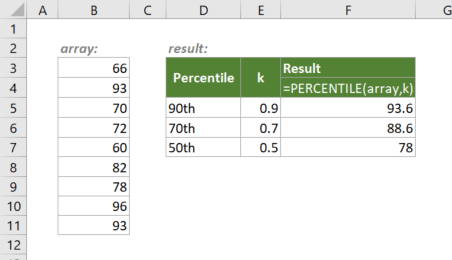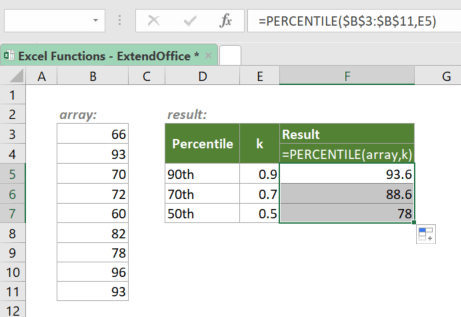## Excel PERCENTILE Function

The Excel PERCENTILE function returns the k-th percentile of the values in a given range. The k is from 0 to 1, including 0 and 1.

Note: PERCENTILE has been replaced with PERCENTILE.INC. Although PERCENTILE is still available in current versions of Excel, you should consider using the new function from now on, because PERCENTILE may not be available in Excel’s future versions.#### Syntax

=PERCENTILE(array, k)

#### Arguments

• array (required): The array or range of data according to which to calculate the k-th percentile.
• k (required): The percentile value or a decimal value in the range from 0 to 1, including 0 and 1. For example, k=0.9 means to the 90th percentile of the values in array, meaning that 90% of values are less than or equal to the calculated result.

#### Return Value

The PERCENTILE function returns a numeric value.

#### Function Notes

• • PERCENTILE returns the #VALUE! error value if k is not numeric.
• • PERCENTILE returns the #NUM! error value if:
• k < 0 or k > 1;
• array is empty.
• If the requested quartile falls between two of the values in the array, PERCENTILE will interpolate and return an intermediate value.

#### Example

To get the 90th, 70th, 50th percentiles of the array as shown below, please copy or enter the formula below in the top cell F5, and press Enter to get the first result:

=PERCENTILE(\$B\$3:\$B\$11,E5)

√ Note: The dollar signs (\$) above indicate absolute references, which means the array argument in the formula won't change when you move or copy the formula to other cells. However, there are no dollar signs added to k since you want it to be dynamic. After entering the formula, drag the fill handle down to apply the formula to the below cells.#### Related functions

Excel PERCENTILE.INC Function

The Excel PERCENTILE.INC function returns the k-th percentile of the values in a given range. The k is from 0 to 1, including 0 and 1.

Excel PERCENTILE.EXC Function

The Excel PERCENTILE.EXC function returns the k-th percentile of the values in a given range. The k is from 1/(n+1) to n/(n+1), including, where n is the number of values in the range.

Excel SMALL Function

The Excel SMALL function returns the k-th smallest value (1st smallest value, 2nd smallest value, etc.) in an array or range of data.

Excel LARGE Function

The Excel LARGE function returns the k-th largest number in an array or range of data.

Excel RANK Function

The RANK function returns the rank of a number compared to other numbers in the same list. The same rank will be assigned to duplicate values in the list.

### The Best Office Productivity Tools

#### Kutools for Excel - Helps You To Stand Out From Crowd

Would you like to complete your daily work quickly and perfectly? Kutools for Excel brings 300 powerful advanced features (Combine workbooks, sum by color, split cell contents, convert date, and so on...) and save 80% time for you.

• Designed for 1500 work scenarios, helps you solve 80% Excel problems.
• Reduce thousands of keyboard and mouse clicks every day, relieve your tired eyes and hands.
• Become an Excel expert in 3 minutes. No longer need to remember any painful formulas and VBA codes.
• 30-day unlimited free trial. 60-day money back guarantee. Free upgrade and support for 2 years.#### Office Tab - Enable Tabbed Reading and Editing in Microsoft Office (include Excel)

• One second to switch between dozens of open documents!
• Reduce hundreds of mouse clicks for you every day, say goodbye to mouse hand.
• Increases your productivity by 50% when viewing and editing multiple documents.
• Brings Efficient Tabs to Office (include Excel), Just Like Chrome, Firefox, And New Internet Explorer.